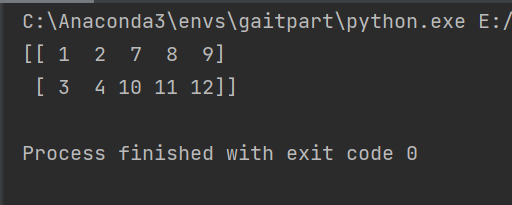# Python如何读取Excel表内容

## 用python读取excel表中的数据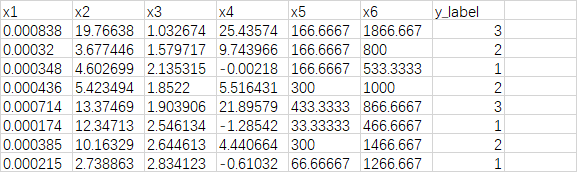``````import pandas as pd

file_path = r'E:\relate_code\svm\dataset\data.xlsx'   # r对路径进行转义，windows需要
print(raw_data)
``````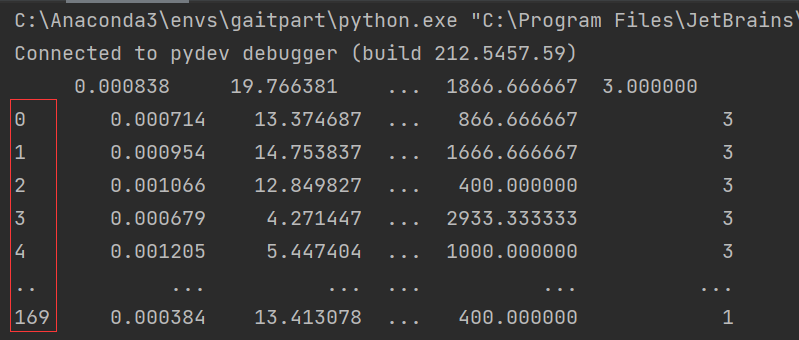``````data = raw_data.value     # 只提取表中信息
print(data)
``````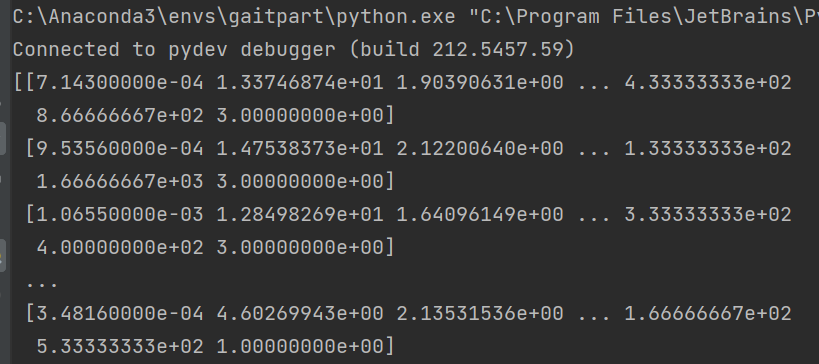``````features = data[:, 0:6]  # 由于是二维数组，所以第一个冒号表示选择所有行，之后0：6表示只要前六列的数据
labels = data[:, -1]     # 标签只要最后一列
``````

1、还可以对特征进行选择，假如只要第四列的特征也可以使用：

``````feature_4 = data[: 3:4]  # 这样得出的数组依然是二维数组，便于后续特征操作
``````

2、如果不想要第四个特征，其它都想要，也可以这样使用，需要用到numpy库：

``````import numpy as np

feature1_3 = data[:, 0:3]   # 取前三列特征
feature5_6 = data[:, 4:6]   # 取第5，第6列特征
feature_choose = np.hstack(feature1_3, feature5_6)   # 对两份特征进行特征拼接
``````

## 这里再多说一下，np.hstack（）函数和 np.vstack（）函数：

``````import numpy as np

arr1 = np.array([[1, 2, 3], [4, 5, 6]])
arr2 = np.array([7, 8, 9])
print(np.vstack((arr1, arr2)))
``````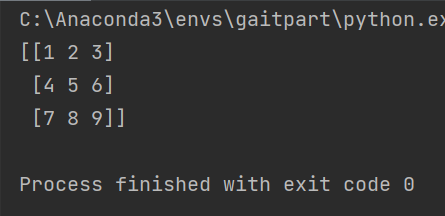``````import numpy as np

arr1 = np.array([[1, 2], [3, 4]])
arr2 = np.array([[7, 8, 9], [10, 11, 12]])
print(np.hstack((arr1, arr2)))
``````